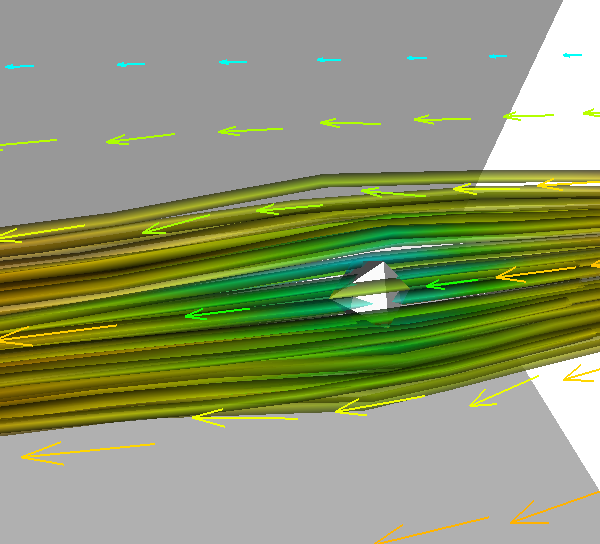VTK, the visualisation toolkit, can be used to view fluid flows in 3-D.

Here are two simple scripts for exporting array information from Matlab into VTK formats, which can then be visualised with software such as Paraview or MayaVi.

## Scalar Data

``````function savevtk(array, filename)
%  savevtk Save a 3-D scalar array in VTK format.
%  savevtk(array, filename) saves a 3-D array of any size to
%  filename in VTK format.
[nx, ny, nz] = size(array);
fid = fopen(filename, 'wt');
fprintf(fid, '# vtk DataFile Version 2.0\n');
fprintf(fid, 'Comment goes here\n');
fprintf(fid, 'ASCII\n');
fprintf(fid, '\n');
fprintf(fid, 'DATASET STRUCTURED_POINTS\n');
fprintf(fid, 'DIMENSIONS    %d   %d   %d\n', nx, ny, nz);
fprintf(fid, '\n');
fprintf(fid, 'ORIGIN    0.000   0.000   0.000\n');
fprintf(fid, 'SPACING    1.000   1.000   1.000\n');
fprintf(fid, '\n');
fprintf(fid, 'POINT_DATA   %d\n', nx*ny*nz);
fprintf(fid, 'SCALARS scalars float\n');
fprintf(fid, 'LOOKUP_TABLE default\n');
fprintf(fid, '\n');
for a=1:nx
for b=1:ny
for c=1:nz
fprintf(fid, '%d ', array(a,b,c));
end
fprintf(fid, '\n');
end
end
fclose(fid);
return``````

## Vector Data

``````function savevtkvector(X, Y, Z, filename)
%  savevtkvector Save a 3-D vector array in VTK format
%  savevtkvector(X,Y,Z,filename) saves a 3-D vector of any size to
%  filename in VTK format. X, Y and Z should be arrays of the same
%  size, each storing speeds in the a single Cartesian directions.
if ((size(X) ~= size(Y)) | (size(X) ~= size(Z)))
fprint('Error: velocity arrays of unequal size\n'); return;
end
[nx, ny, nz] = size(X);
fid = fopen(filename, 'wt');
fprintf(fid, '# vtk DataFile Version 2.0\n');
fprintf(fid, 'Comment goes here\n');
fprintf(fid, 'ASCII\n');
fprintf(fid, '\n');
fprintf(fid, 'DATASET STRUCTURED_POINTS\n');
fprintf(fid, 'DIMENSIONS    %d   %d   %d\n', nx, ny, nz);
fprintf(fid, '\n');
fprintf(fid, 'ORIGIN    0.000   0.000   0.000\n');
fprintf(fid, 'SPACING    1.000   1.000   1.000\n');
fprintf(fid, '\n');
fprintf(fid, 'POINT_DATA   %d\n', nx*ny*nz);
fprintf(fid, 'VECTORS vectors float\n');
fprintf(fid, '\n');
for a=1:nx
for b=1:ny
for c=1:nz
fprintf(fid, '%f ', X(a,b,c));
fprintf(fid, '%f ', Y(a,b,c));
fprintf(fid, '%f ', Z(a,b,c));
end
fprintf(fid, '\n');
end
end
fclose(fid);
return``````

With those, some data, and VTK-based software, you can easily create images like this:Fluid flow as imaged by VTK using MayaVi, with data exported from Matlab.